The K5 Learning Blog urges parents to be pro-active in helping their children reach their full academic potential.

# How to Find the Area of Irregular Shapes

Faced with an irregular shape, and asked to work out its surface area, can be daunting to students. As with more complex math problems, it’s best to break this problem down in steps.

Let’s use an example. Work out the area of this shape.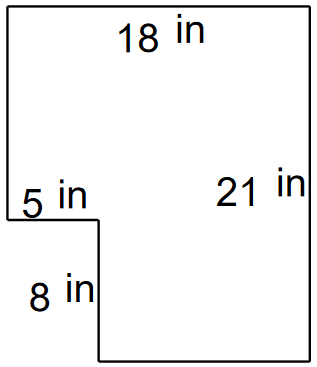It does look daunting, doesn’t it? So, let’s break it into steps.

This irregular shape is made of rectangles. How, do you say? We break it into its two rectangular shapes.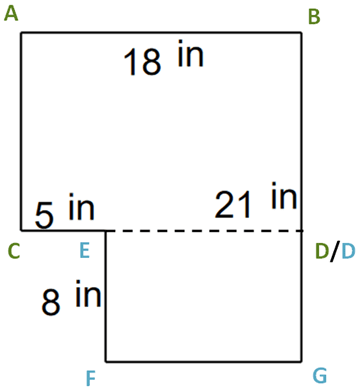Now we have two rectangles.

The first rectangle is ABCD
A - B, / C - D is 18 inches long.
A – C / B – D is 21 – 8 inches long.

Let’s explain that. The shape does not indicate how long B - D is. However, we have:

B - G = 21 inches
and
D – G = 8 inches

To work out B - D, we need to subtract D – G from B – G:
21 – 8 = 13

The area of ABCD = 13 x 18 = 234 square inches.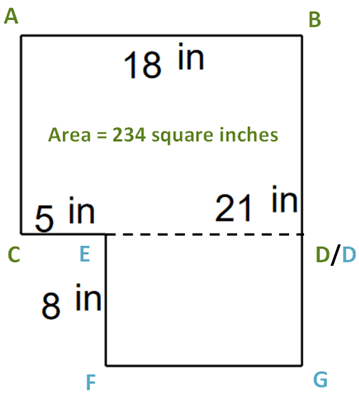Now, let’s turn our attention to the second rectangle: EDFG.

Again, we need to do some subtraction:
E – D / F – G is not indicated, but we have C – D and C – E.

To work our E – D, we subtract C – E from C – D.
18 – 5 = 13

Area EDFG = 8 x 13 = 104 square inches.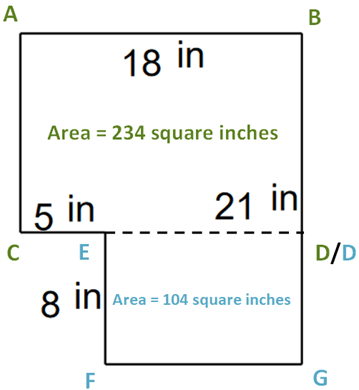Finally, we add the two areas to work out the area of the irregular shape:

234 + 104 = 338 square inches.

## Grade 5 irregular shapes worksheets

In our grade 5 geometry section we have worksheets to practice both the area and the perimeter of irregular rectangular shapes.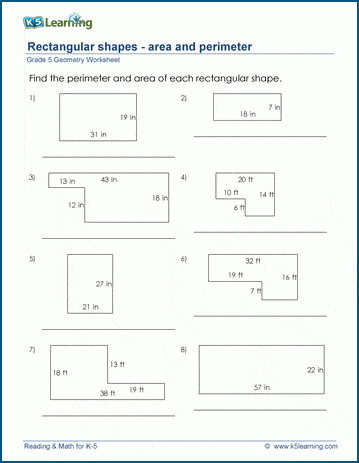## Grade 6 irregular rectangular shapes worksheets

We have further practice to work out the area and perimeter of irregular shapes in our grade 6 geometry section.